##### Basic Math & Pre-Algebra All-in-One For Dummies (+ Chapter Quizzes Online)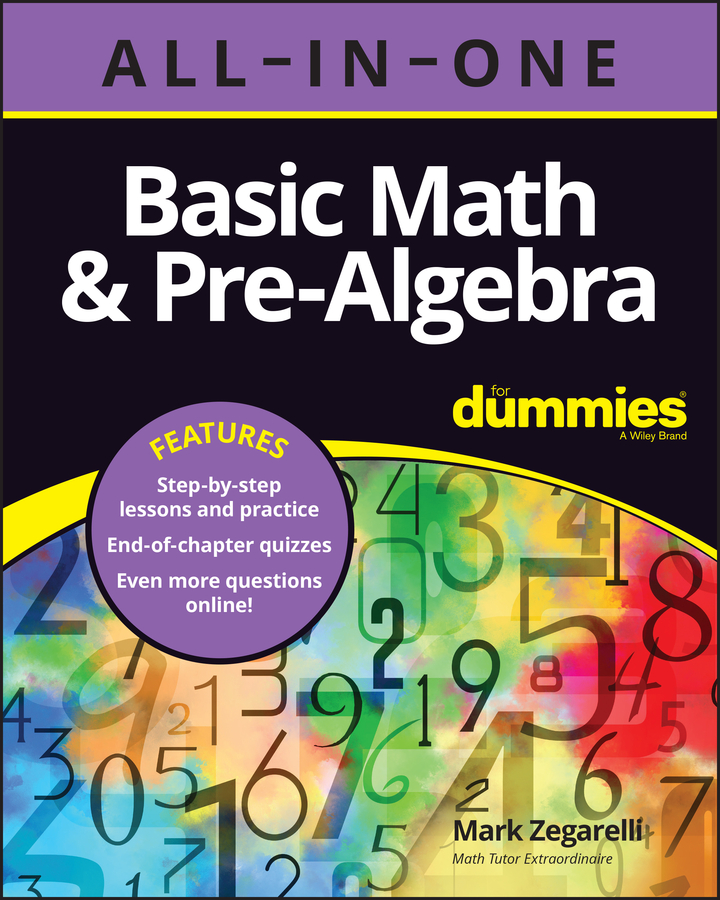Probability is the mathematics of deciding how likely an event is to occur. It has a wide variety of applications in insurance, weather prediction, biological sciences, and even physics. For example,
• What’s the likelihood that the lottery ticket I bought will win?

• What’s the likelihood that my new car will need repairs before the warranty runs out?

• What’s the likelihood that more than 100 inches of snow will fall in Manchester, New Hampshire, this winter?

The probability that an event will occur is a fraction whose numerator (top number) and denominator (bottom number) are as follows: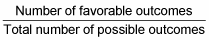In this case, a favorable outcome is simply an outcome in which the event you’re examining does happen. In contrast, a possible outcome is an outcome that can happen.

For example, suppose you want to know the probability that a tossed coin will land heads up. Notice that there are two possible outcomes (heads or tails), but only one of these outcomes is favorable — the outcome in which heads comes up. To find the probability of this event, make a fraction as follows: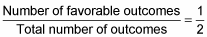So the probability that the coin will land heads up is 1/2.

So what’s the probability that when you roll a die, the number 3 will land face up? To figure this one out, notice that there are six possible outcomes (1, 2, 3, 4, 5, or 6), but in only one of these does 3 land face up. To find the probability of this outcome, make a fraction as follows: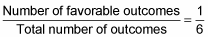So the probability that the number 3 will land face up is 1/6.

And what’s the probability that if you pick a card at random from a deck, it’ll be an ace? To figure this out, notice that there are 52 possible outcomes (one for each card in the deck), but in only four of these do you pick an ace. So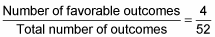So the probability that you’ll pick an ace is 4/52, which reduces to 1/13.

Probability is always a fraction or decimal from 0 to 1. When the probability of an outcome is 0, the outcome is impossible. When the probability of an outcome is 1, the outcome is certain.